# Orthocenter

Orthocenter

The term "ortho" means "right." Hence, orthocenter indicates the center of all the right angles from the vertices to the opposite sides i.e., the altitudes.

This concept has significant importance in the study of the various properties of a triangle with respect to its other dimensions.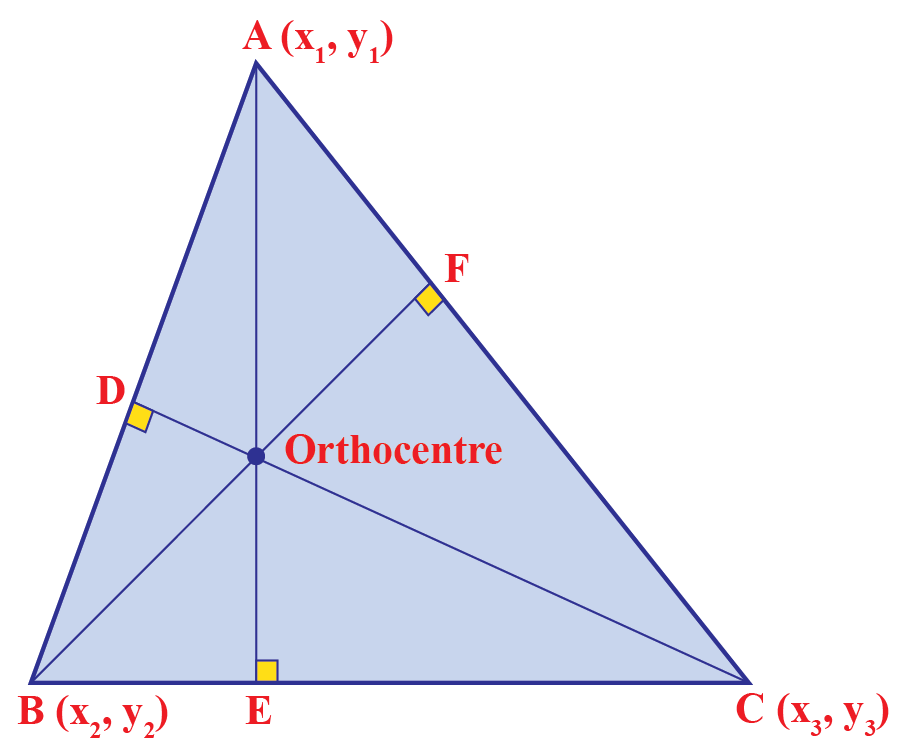In this chapter, you will learn about orthocenter properties, orthocenter formula, orthocenter definition, and the orthocenter of a right triangle in the concept of the orthocenter.

Check out the interactive simulation to know more about the lesson and try your hand at solving a few interesting practice questions at the end of the page.

## Lesson Plan

 1 What Is an Orthocenter? 2 Important Notes on Orthocenter 3 Challenging Questions on Orthocenter 4 Solved Examples 5 Interactive Questions

## What Is an Orthocenter?

### Definition

The orthocenter is that point where all the three altitudes of a triangle intersect.### Triangle

A polygon with three vertices and three edges is called a triangle.

In the above figure, $$\bigtriangleup$$ABC is a triangle.

### Altitude

The altitude of a triangle is that line that passes through its vertex and is perpendicular to the opposite side. Hence, a triangle can have three altitudes, one from each vertex.

In the above figure, $$\bigtriangleup$$ABC has three altitudes, namely, $$\text {AE, BF, and, CD}$$.

### Vertex

The point where two or more lines meet is called a vertex.

In the above figure, $$\bigtriangleup$$ABC has three vertices, namely, $$\text {A, B, and, C}$$.

## What Is the General Formula for the Orthocenter of a Triangle?

The orthocenter formula helps in locating the coordinates of the orthocenter of a triangle.

Let us consider a triangle PQR, as shown in the figure below.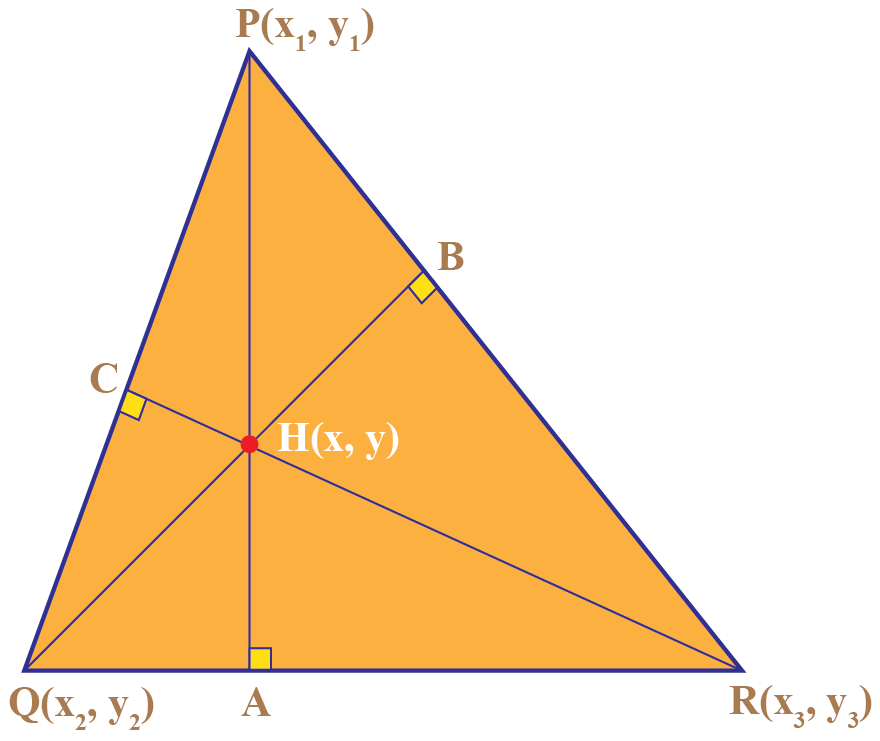PA, QB, RC are the perpendicular lines drawn from the three vertices P($$x_{1}, y_{1})$$, Q($$x_{2}, y_{2})$$, and R($$x_{3}, y_{3})$$ respectively of the $$\bigtriangleup$$PQR.

H$$( x, y)$$ is the intersection point of the three altitudes of the triangle.

Step1. Calculate the slope of the sides of the triangle using the formula:

m(slope) = $$\frac{y_{2} \ - \ y_{1}}{x_{2} \ - \ x_{1}}$$

Let slope of PR be given by mPR.

Hence,

mPR = $$\frac{ y_3 \ - \ y_1 }{ x_3 \ - \ x_1 }$$

Similarly,

mQR = $$\frac{ y_3 \ - \ y_2 }{ x_3 \ - \ x_2 }$$

Step2. The slope of the altitudes of the $$\bigtriangleup$$PQR will be perpendicular to the slope of the sides of the triangle.

We know,

\begin{align*} \text {Perpendicular slope of line} \ &= \ \frac{-1}{ \text {slope of the line}} \\ &= \frac{-1}{ \text m} \end{align*}

The slope of the respective altitudes:

Slope of PA, mPA = $$\frac{-1}{ \text {mQR}}$$

Slope of QB, mQB = $$\frac{ -1}{ \text {mPR}}$$

We will use the slope-point form equation as a straight line to calculate the equations of the lines coinciding with PA and QB.

The generalized equation thus formed by using arbitrary points $$x$$ and $$y$$ is:

\begin{align*} \text {mPA} &= \frac{( y \ - \ y_1 )}{( x \ - \ x_1 )} \\ \text {mQB} &= \frac{( y \ - \ y_2)}{( x \ - \ x_2 )} \end{align*}

Thus, solving the two equations for any given values the orthocenter of a triangle can be calculated.

## What Are the Properties of the Orthocenter?

The various properties of the orthocenter are:

1. The orthocenter of an acute triangle lies inside the triangle.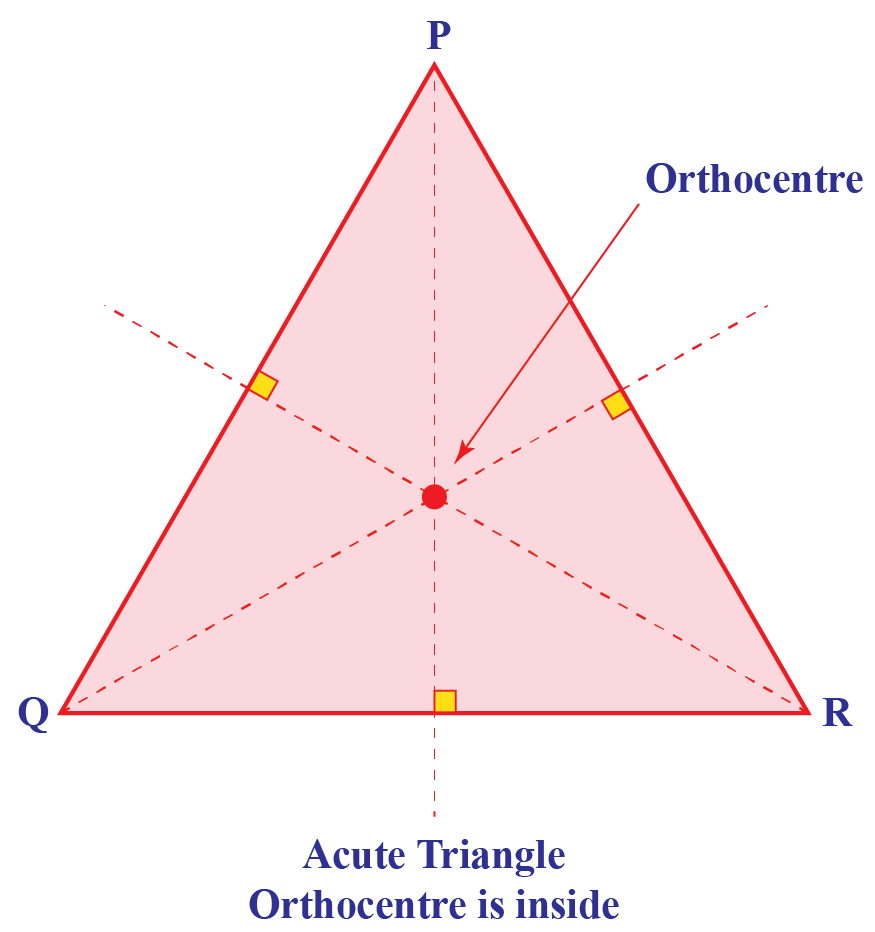2. The orthocenter of an obtuse triangle lies outside the triangle.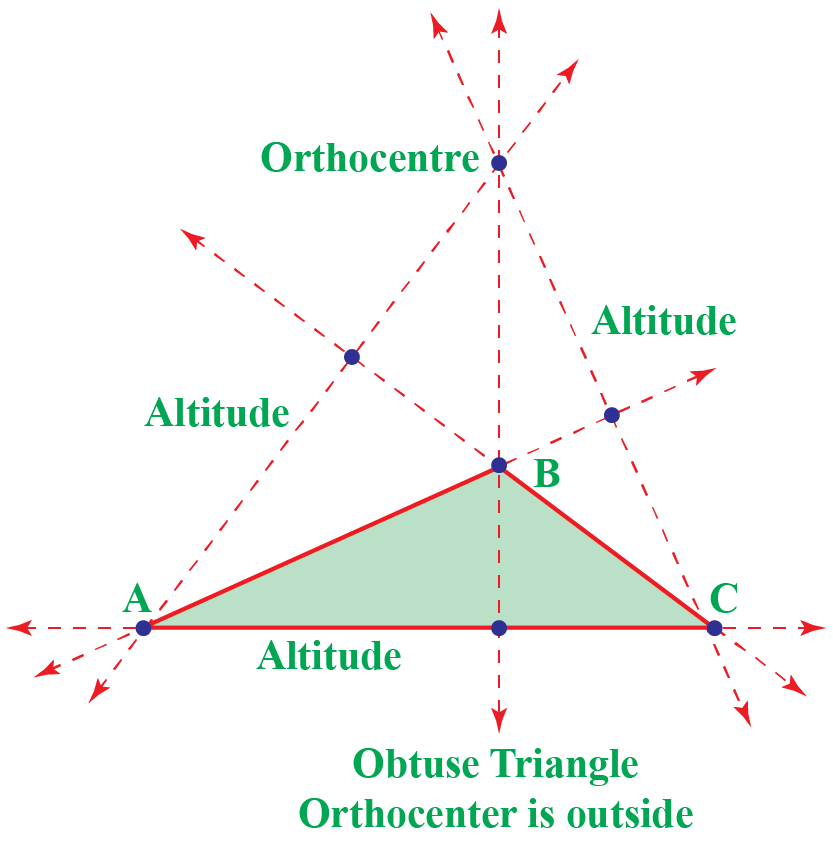3. The orthocenter of a right-angled triangle lies on the vertex of the right angle.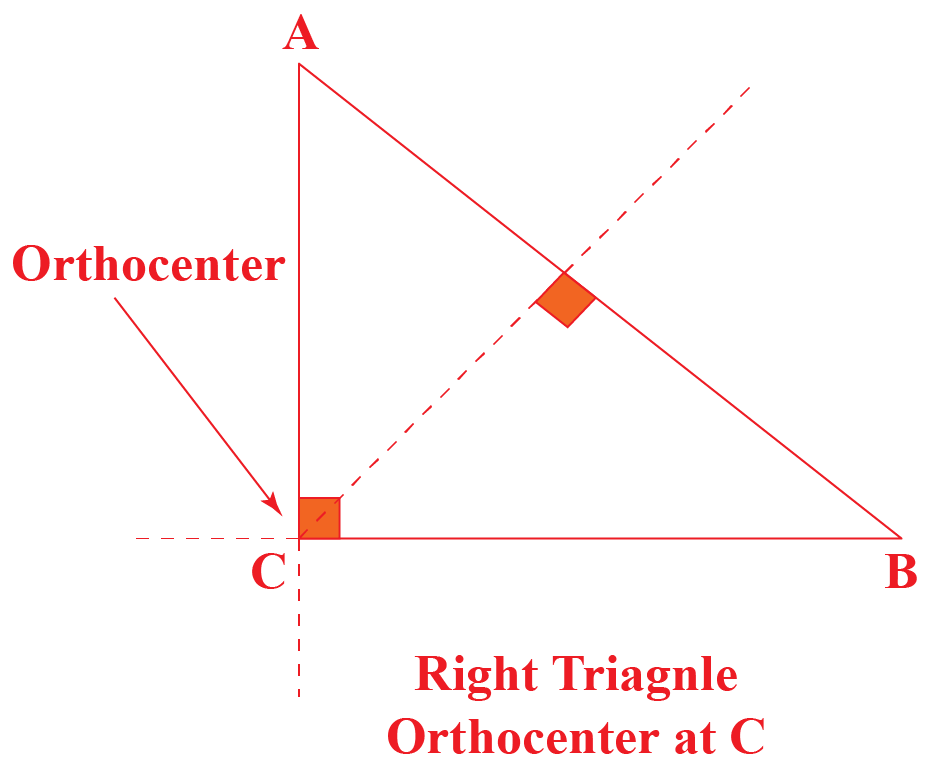4. An orthocenter divides an altitude into different parts. The product of the lengths of all these parts is equivalent for all the three perpendiculars.

## How to Construct an Orthocenter?

To construct the orthocenter for a triangle geometrically, we have to do the following:

• Find the perpendicular from any two vertices to the opposite sides.
• To draw the perpendicular or the altitude, use vertex C as the center and radius equal to the side BC. Draw arcs on the opposite sides AB and AC.
Draw intersecting arcs from B and D, at F. Join CF.
Similarly, draw intersecting arcs from points C and E, at G. Join BG.
CF and BG are altitudes or perpendiculars for the sides AB and AC respectively.
• The intersection point of any two altitudes of a triangle gives the orthocenter.
Thus, find the point of intersection of the two altitudes.
• At that point, H is referred to as the orthocenter of the triangle.

Refer to the visual demonstration of how to construct an orthocenter in the following simulation:

## Solved Examples

 Example 1

Can you help Sam name the vertices, sides, altitudes, and orthocenter for the following figure?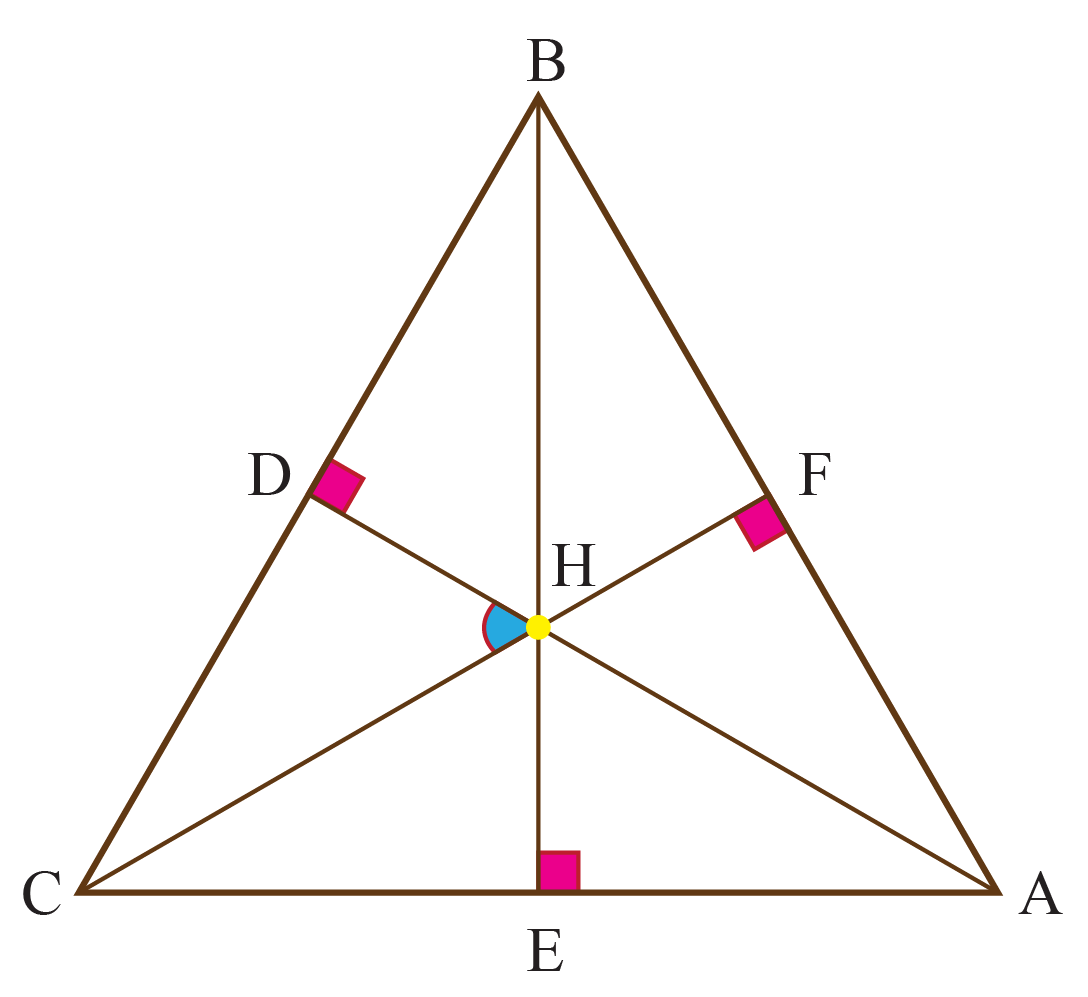Solution

In the above figure for $$\triangle{ABC}$$,

Vertices: A, B, and C

Sides: AB, BC, AC

Altitudes: AD, BE, CF

Orthocenter: H

 Example 2

Point H is the orthocenter of $$\triangle{ABC}$$.

If $$\angle{B} \ = \ 58^\circ , \ \angle{C}=60^\circ , \ \text {and} \angle{A}=62^\circ$$. What is the measure of $$\angle{CHD}$$?Solution

In the given figure,

\begin{align*} \angle{ \text{CDH}} &= \angle{ \text{BFC}} = 90^\circ \\ \angle{ \text{DCH}} &= \angle{ \text {BCF}} \end{align*}

Hence, by AAA property,

$$\triangle{ \text {DCH}}$$ and $$\triangle{ \text {FCB}}$$ are similar triangles.

Thus,

$$\angle{ \text {CHD}} = \angle{ \text {CBF}} = 58^\circ$$

 $$\therefore$$ $$\angle{ \text {CHD}} = 58^\circ$$
 Example 3

Can you help Emma find the slopes of the altitudes of $$\triangle{ \text {ABC}}$$ when its vertices are A (-5, 3), B (1, 7), C (7, -5)?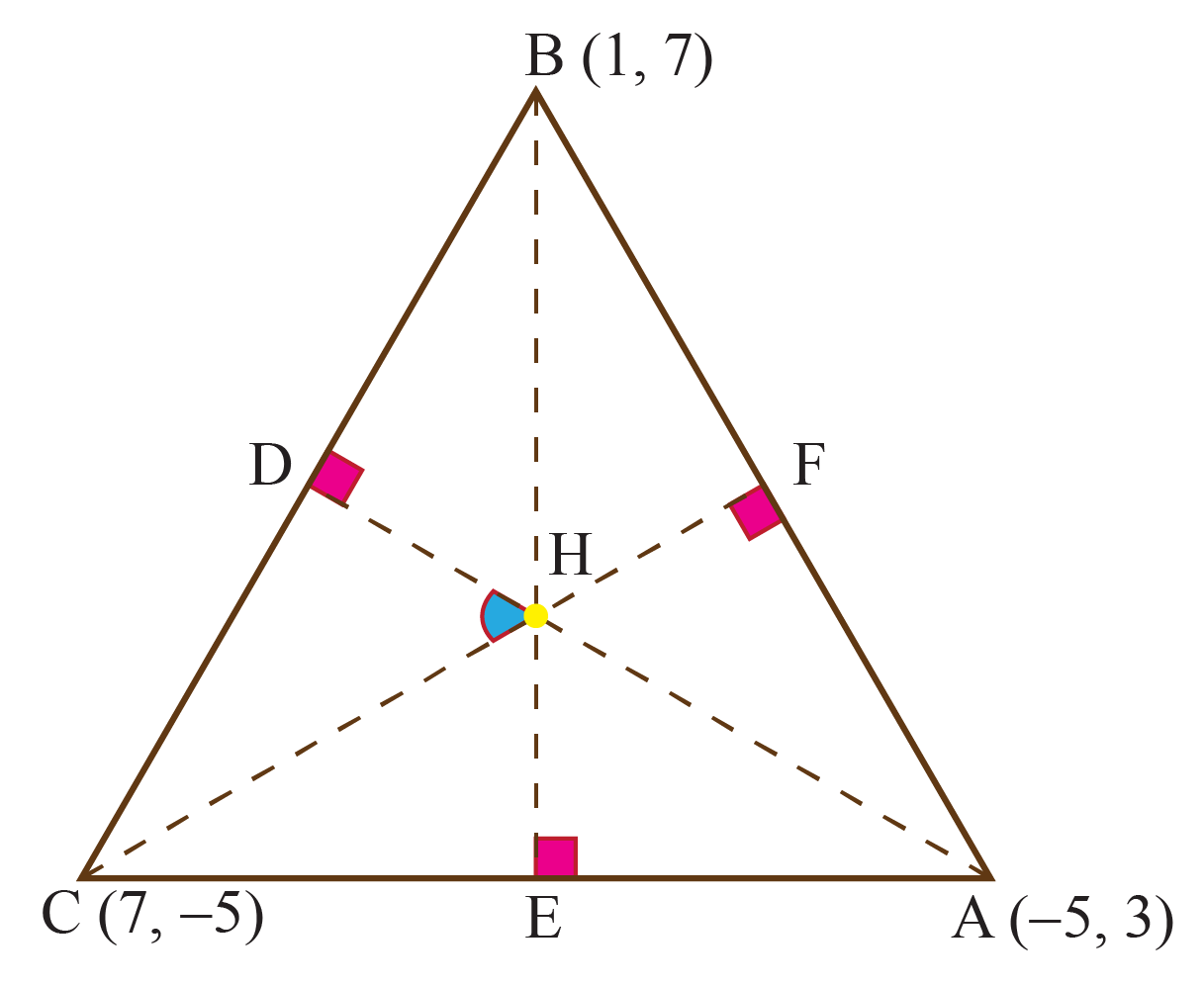Solution

Given, the vertices of the triangle,

\begin{align*} \text A &= (-5, 3) \\ \text B &= (1, 7) \\ \text C &= (7, -5) \end{align*}

Slope of AB,

\begin{align*} \text {mAB} &= \frac{y_{2} \ - \ y_{1}}{x_{2} \ - \ x_{1}} \\ &= \frac{7 - 3}{1 + 5} \\ &= \frac{4}{6} \\ &= \frac{2}{3} \end{align*}

Slope of CF,

\begin{align*} \text {mCF} &= \text {Perpendicular slope of AB} \\ &= \frac{-1}{ \text {mAB}} \\ &= \frac{-3}{2} \end{align*}

Slope of BC,

\begin{align*} \text {mBC} &= \frac{y_{2} \ - \ y_{1}}{x_{2} \ - \ x_{1}} \\ &= \frac{-5 - 7}{7 - 1} \\ &= \frac{-12}{6} \\ &= -2 \end{align*}

Slope of AD,

\begin{align*} \text {mAD} &= \text {Perpendicular slope of BC} \\ &= \frac{-1}{ \text {mBC}} \\ &= \frac{1}{2} \end{align*}

Slope of AC,

\begin{align*} \text {mAC} &= \frac{y_{2} \ - \ y_{1}}{x_{2} \ - \ x_{1}} \\ &= \frac{-5 - 3}{7 + 5} \\ &= \frac{-8}{12} \\ &= \frac{-2}{3} \end{align*}

Slope of BE,

\begin{align*} \text {mBE} &= \text {Perpendicular slope of AC} \\ &= \frac{-1}{ \text {mAC}} \\ &= \frac{3}{2} \end{align*}

 $$\therefore \frac{-3}{2} , \frac{1}{2} , \frac{3}{2}$$
 Example 4

Construct the orthocenter of a triangle with sides measuring 5 inches, 5.5 inches, and 6 inches.

Solution

Steps of Construction:

• Find the perpendicular from any two vertices to the opposite sides.
• To draw the perpendicular or the altitude, refer to section- How to Construct anthocOrenter?
• Draw altitude for any two sides, here the ones for AB and BC are drawn.
• The intersection point of any two altitudes of a triangle gives the orthocenter.
Thus, find the point of intersection of the two altitudes.
• At that point, H is referred to as the orthocenter of the triangle.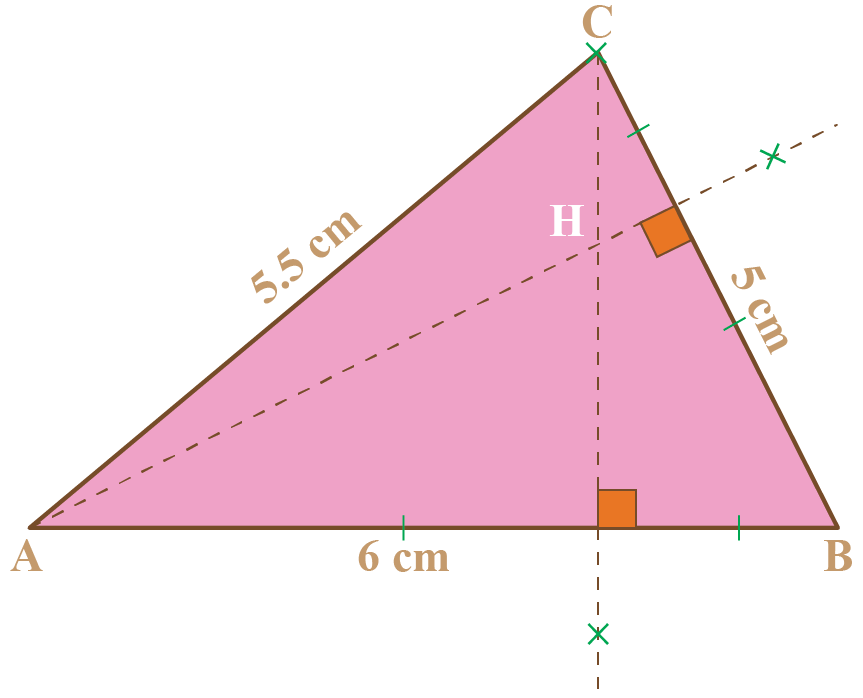$$\therefore$$ H is the orthocenter for the $$\triangle{ \text {ABC}}$$
 Example 5

Can you help Kevin find the orthocenter of a triangle when their vertices are A(1, 3), B(2, 5), C(3, -4).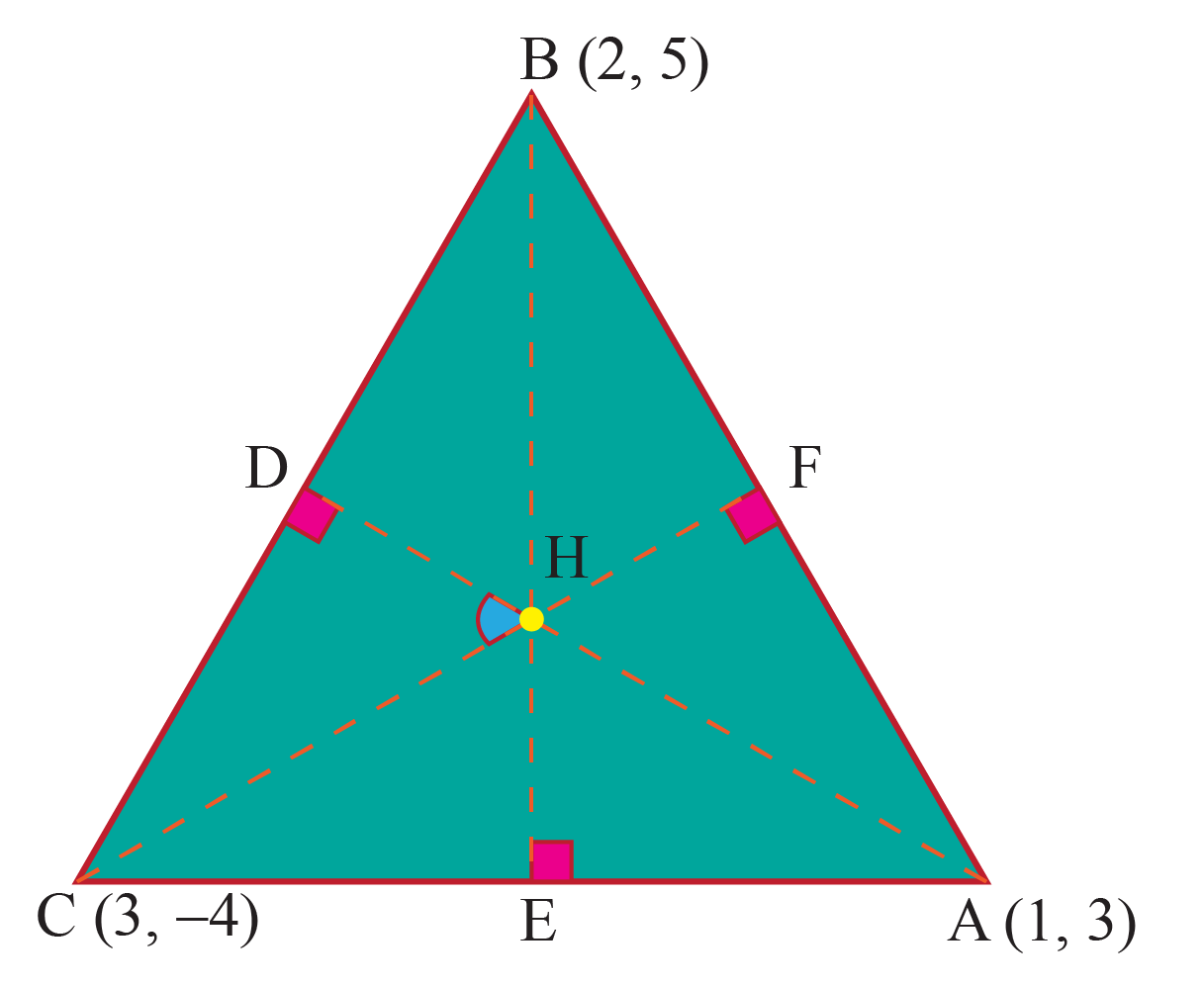Solution

Given, the vertices of the triangle,

\begin{align*} \text A &= (1, 3) \\ \text B &= (2, 5) \\ \text C &= (3, -4) \end{align*}

Let the coordinates of orthocenter be H(x, y).

Slope of AB,

\begin{align*} \text {mAB} &= \frac{( y_{2} \ - \ y_{1})}{( x_{2} \ - \ x_{1})} \\ &= \frac{5 - 3}{2 - 1} \\ &= \ 2 \end{align*}

Slope of CF,

\begin{align*} \text {mCF} &= \text {Perpendicular slope of AB} \\ &= \frac{-1}{ \text {mAB}} \\ &= \frac{-1}{2} \end{align*}

The equation of CF is given as,

\begin{align*} ( y – \text y_1) &= \text m ( x – \text x_1) \\ y + 4 &= \frac{-1}{2} ( x – 3) \\ 2 y + 8 &= - x + 3 \\ x + 2 y &= -5 \ \ \ \ ( \text {equation} 1) \end{align*}

Slope of BC,

\begin{align*} \text {mBC} &= \frac{(y_{2} \ - \ y_{1})}{(x_{2} \ - \ x_{1})} \\ &= \frac{-4 - 5}{3 - 2} \\ &= -9 \end{align*}

Slope of AD,

\begin{align*} \text {mAD} &= \text {Perpendicular slope of BC} \\ &= \frac{-1}{ \text {mBC}} \\ &= \frac{-1}{-9} \\ &= \frac{1}{9} \end{align*}

The equation of AD is given as,

\begin{align*} ( y – \text y_1) &= \text m ( x – x_1) \\ y - 3 &= \frac{1}{9} ( x – 1) \\ 9 y - 27 &= x - 1 \\ x - 9 y &= -26 \ \ \ \ ( \text {equation} 2)\end{align*}

Subtracting equation (2) and (1),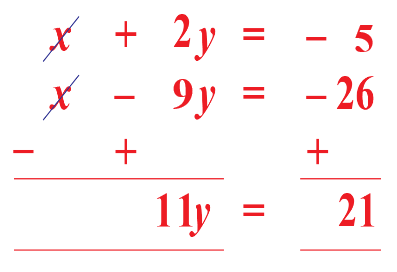$y = \dfrac{21}{11}$

Thus, putting this value in equation (1),

$x = -\dfrac {97}{11}$

 $$\therefore$$ Orthocenter = $$\left(-\dfrac {97}{11}, \dfrac{21}{11}\right)$$Challenging Questions
• What is the distance between the orthocenter and the circumcenter of a triangle whose sides measure 23 inches, 26 inches, and 8 inches?
• A straight line 4x + 5y = 26 forms a triangle with the coordinate axes. What is the distance between the orthocenter of the triangle and the center of the circle that circumscribes the triangle?
• How would you differentiate between the orthocenter, circumcenter, incenter, and centroid of a triangle?

## Interactive Questions

Here are a few activities for you to practice. Select/Type your answer and click the "Check Answer" button to see the result.

## Let's Summarize

The mini-lesson targeted the fascinating concept of the Orthocenter. The math journey around the Orthocenter started with what a student already knew and went on to creatively crafting a fresh concept in the young minds. Done in a way that not only it is relatable and easy to grasp, but also will stay with them forever.

## About Cuemath

At Cuemath, our team of math experts is dedicated to making learning fun for our favorite readers, the students!

Through an interactive and engaging learning-teaching-learning approach, the teachers explore all angles of a topic.

Be it worksheets, online classes, doubt sessions, or any other form of relation, it’s the logical thinking and smart learning approach that we, at Cuemath, believe in.

## 1. Are orthocenter and circumcenter the same?

No, the orthocenter and circumcenter of a triangle are different.

The orthocenter of a triangle is the point of intersection of all the three altitudes drawn from the vertices of a triangle to the opposite sides.

The circumcenter of a triangle is the point of intersection of the perpendicular bisector of the three sides.

## 2. Why is it called an orthocenter?

The term "ortho" means "right" and center means the midpoint. Thus, clubbing the two words together here means center for the altitudes (right angles) of the triangle.

Hence, it is called an orthocenter.

## 3. Why is orthocenter important?

The orthocenter of a triangle is the point of intersection of all the three altitudes drawn from the vertices of a triangle to the opposite sides.

It is an important central point of a triangle and thus helps in studying different properties of a triangle with respect to sides, vertices, other important points like circumcenter, centroid, etc.

More Important Topics
Numbers
Algebra
Geometry
Measurement
Money
Data
Trigonometry
Calculus